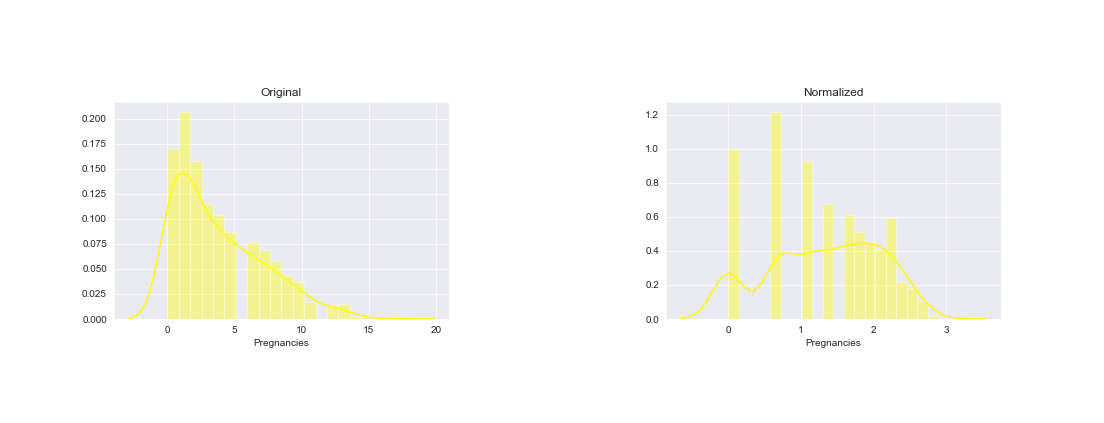# Bring your data to normal distribution with imperio YeoJohnsonTransformer

One of the biggest problems with data in Data Science is its distribution, it almost every single time isn’t normal. It happens because we cannot have all samples in the world in one data set. However, there exists a bunch of methods that can change that. In one of the previous articles, we looked at Box-Cox transformation. Today we will take a look at the Yeo-Johnson transformation.

## HowYeo-Johnson transformation works?

Yeo-Johnson transformation is a transformation of a non-normal variable into a normal one, following the same aim as Box-Cox. However, Yeo-Johnson is going even further, allowing y to have 0 and even negative values. In the Box-Cox article, we said that the normality of the data is a very important assumption in statistics. From the point of view of statistics, it allows you to run more statistics tests on the data, while from the Machine Learning point of view it allows algorithms easier to learn.
Yeo-Johnson applies the following formula to the data:

This formula gives the following effect:The pregnacies column distribution from Pima Diabetes data set before and after applying Yeo-Johnson.

## Using imperio YeoJohnsonTransformer:

All transformers from imperio follow the transformers API from sci-kit-learn, which makes them fully compatible with sci-kit learn pipelines. First, if you didn’t install the library, then you can do it by typing the following command:

``````pip install imperio
``````

Now you can import the transformer, fit it and transform some data.

``````from imperio import YeoJohnsonTransformer
yeo_johnson = YeoJohnsonTransformer()
yeo_johnson.fit(X_train, y_train)
X_trainsformed = yeo_johnson.transform(X_test)
``````

Also, you can fit and transform the data at the same time.

``````X_transformed = yeo_johnson.fit_transform(X_train, y_train)
``````

As we said it can be easily used in a sci-kit learn pipeline.

``````from sklearn.pipeline import Pipeline
from imperio import YeoJohnsonTransformer
from sklearn.linear_model import LogisticRegression
pipe = Pipeline(
[
('yeo_johnson', YeoJohnsonTransformer()),
('model', LogisticRegression())
])
``````

Besides the sci-kit learn API, Imperio transformers have an additional function that allows the transformed to be applied on a pandas data frame.

``````new_df = yeo_johnson.apply(df, target = 'target', columns=['col1'])
``````

The YeoJohnsonTransformer constructor has the following arguments:

• l (float, default = 0.5): The lambda parameter used by Yeo-Johnson Algorithm to choose the transformation applied to the data.
• index (list, default = None): A parameter that specifies the list of indexes of numerical columns that should be transformed.

The apply function has the following arguments.

• df (pd.DataFrame): The pandas DataFrame on which the transformer should be applied.
• target (str): The name of the target column.
• columns (list, default = None): The list with the names of columns on which the transformers should be applied.

Now let’s apply it to a data set. We will use it on the Pima diabetes dataset. We will apply it to all columns, however, we recommend applying it only on the numerical columns. Also in this case we will apply a Standard Scaler. Bellow, you can see the Logistics Regression performance before applying the Yeo-Johnson transformer and after applying it.

As we can see the accuracy of the Logistic Regression model raised from 0.72 to 0.74 after applying a distribution normalizer to our data.